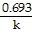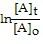# quiz 2 Flashcards

Set Details Share
created 7 years ago by jkarlowicz123
3,892 views
Page to share:
Embed this setcancel
COPY
code changes based on your size selection
Size:
X

1

Choose the molecule or compound that exhibits dipole-dipole forces as its strongest intermolecular force.

SO2

H2

CF4

BCl3

NH3

SO2

2

Choose the pair of substances that are most likely to form a homogeneous solution.

LiF and C6H14

C3H8 and C2H5OH

Br2 and PF3

NH3 and CH3OH

NaCl and Hg

NH3 and CH3OH

3

Choose the substance with the highest boiling point.

CS2

HF

I2

KI

CH4

KI

4

Choose the substance with the lowest surface tension.

CH3CH2CH2CH3

H2O

C6H6

(CH3)2CO

CH3SH

CH3CH2CH2CH3

5

Choose the substance with the lowest vapor pressure at a given temperature.

He

BF3

BeCl2

CO2

PF5

BeCl2

6

Choose the substance with the lowest viscosity.

Cl2CHCH2Cl

Cl3CCCl3

Cl3CCHCl2

ClCH2CH2Cl

Cl2CHCHCl2

ClCH2CH2Cl

7

Give the change in condition to go from a liquid to a gas.

Cool or reduce pressure

Increase heat or reduce pressure

Cool or increase pressure

Increase heat or increase pressure

None of the above

Increase heat or reduce pressure

8

Give the characteristic of a first order reaction having only one reactant.

The rate of the reaction is directly proportional to the concentration of the reactant.

The rate of the reaction is proportional to the natural logarithm of the concentration of the reactant.

The rate of the reaction is proportional to the square root of the concentration of the reactant.

The rate of the reaction is not proportional to the concentration of the reactant.

The rate of the reaction is proportional to the square of the concentration of the reactant.

The rate of the reaction is directly proportional to the concentration of the reactant.

9

Give the term for the temperature at which the gas and liquid phases form a supercritical fluid.

Definite temperature.

Absolute temperature.

Critical temperature.

Fluid temperature.

Solid temperature

Critical temperature.

10

Given the following balanced equation, determine the rate of reaction with respect to [NOCl]. If the rate of Cl2 loss is 4.84 × 10-2 M/s, what is the rate of formation of NOCl?
2 NO(g) + Cl2(g) → 2 NOCl(g)

4.84 × 10-2 M/s

1.61 × 10-2 M/s

1.45 × 10-1 M/s

9.68 × 10-2 M/s

2.42 × 10-2 M/s

9.68 × 10-2 M/s

11

Given the following rate law, how does the rate of reaction change if the concentration of X is halved and the concentration of Y is doubled?
Rate = k [X][Y]2

The rate of reaction will increase by a factor of 5.

The rate of reaction will increase by a factor of 2.

The rate of reaction will decrease by a factor of 2.

The rate of reaction will increase by a factor of 4.

The rate of reaction will remain unchanged.

The rate of reaction will increase by a factor of 2.

12

How many half-lives are required for the concentration of reactant to decrease to 12.5% of its original value?

2

1.75

3

2.75

1

3

13

How much energy is required to vaporize 48.7 g of dichloromethane (CH2Cl2) at its boiling point, if its ΔHvap is 31.6 kJ/mol?

18.1 kJ

55.1 kJ

31.2 kJ

15.4 kJ

6.49 kJ

18.1 kJ

14

Place the following compounds in order of decreasing strength of intermolecular forces.
HF O2 CO2

HF > O2 > CO2

O2 > CO2 > HF

CO2 > HF > O2

HF > CO2 > O2

CO2 > O2 > HF

HF > CO2 > O2

15

Place the following compounds in order of increasing strength of intermolecular forces.
CO2 F2 NH2CH3

NH2CH3 < F2 < CO2

NH2CH3 < CO2 < F2

F2 < CO2 < NH2CH3

CO2 < NH2CH3 < F2

F2 < NH2CH3 < CO2

F2 < CO2 < NH2CH3

16

What are the units of k in a first order reaction?

1/s

17

What are the units of k in the following rate law?
Rate = k[X][Y]

M-1s-1

18

What data should be plotted to show that experimental concentration data fits a second-order reaction?

1/[reactant] vs. time

ln[reactant] vs. time

ln(k) vs. Ea

ln(k) vs. 1/T

[reactant] vs. time

1/[reactant] vs. time

19

What is the overall order of the following reaction, given the rate law?
2 X + 3 Y → 2 Z Rate = k[X]1[Y]2

1st order

0th order

2nd order

5th order

3rd order

3rd order

20

What is the overall order of the following reaction, given the rate law?
2NO(g) + H2(g) → N2(g) + 2H2O(g) Rate = k[NO]2[H2]

0th order

3rd order

4th order

2nd order

1st order

3rd order

21

What is the strongest type of intermolecular force present in CHF3?

hydrogen bonding

ion-dipole

dipole-dipole

dispersion

None of the above

dipole-dipole

22

What is the strongest type of intermolecular force present in H2?

dispersion

hydrogen bonding

ion-dipole

dipole-dipole

None of the above

dispersion

23

What is the strongest type of intermolecular force present in NH2CH3?

dipole-dipole

ion-dipole

dispersion

hydrogen bonding

None of the above

hydrogen bonding

24

represents the equation for a first-order half-life?t 1/2 =

25

represents the integrated rate law for a first-order reaction?= -kt

26

Which of the following statements is TRUE?

Until a certain point, the potential energy of molecules decrease as they get closer to one another.

Intermolecular forces are generally stronger than bonding forces.

Increasing the pressure on a solid usually causes it to become a liquid.

Energy is given off when the attraction between two molecules is broken.

None of the above are true.

Until a certain point, the potential energy of molecules decrease as they get closer to one another.

27

Which substance below has the strongest intermolecular forces?

C3X2, ΔHvap= 36.4 kJ/mol

DX2, ΔHvap= 23.3 kJ/mol

BY2, ΔHvap= 26.7 kJ/mol

A2X, ΔHvap= 39.6 kJ/mol

EY3, ΔHvap= 21.5 kJ/mol

A2X, ΔHvap= 39.6 kJ/mol